Courses

# Osmosis and Osmotic Pressure Class 12 Notes | EduRev

## Class 12 : Osmosis and Osmotic Pressure Class 12 Notes | EduRev

The document Osmosis and Osmotic Pressure Class 12 Notes | EduRev is a part of the Class 12 Course Chemistry for JEE.
All you need of Class 12 at this link: Class 12

What is Osmosis?

You haven't seen pickles being made at home, have you? That's all there is to osmosis' magic. How does osmosis work, though? Do you realise that osmosis is one of the most important factors in the survival of many species? We will learn everything about osmosis and osmotic pressure in this document.

In the process of Osmosis, solvent moves from a region of its higher concentration to a region of its lower concentration through a semi-permeable membrane, which allows the free passage of only solvent molecules.

Osmosis is the phenomenon of solvent flow through a semi-permeable membrane to equalize the solute concentrations on both sides of the membrane.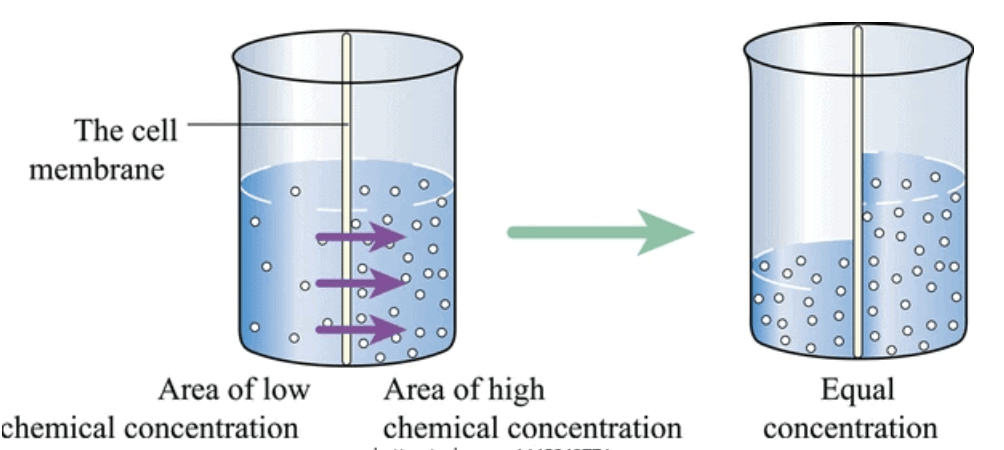What is Osmotic Pressure?

Osmotic Pressure can be defined as:

The minimum pressure which should be applied on the solution, so as to prevent the migration of solvent molecules into the solution through a semi-permeable membrane.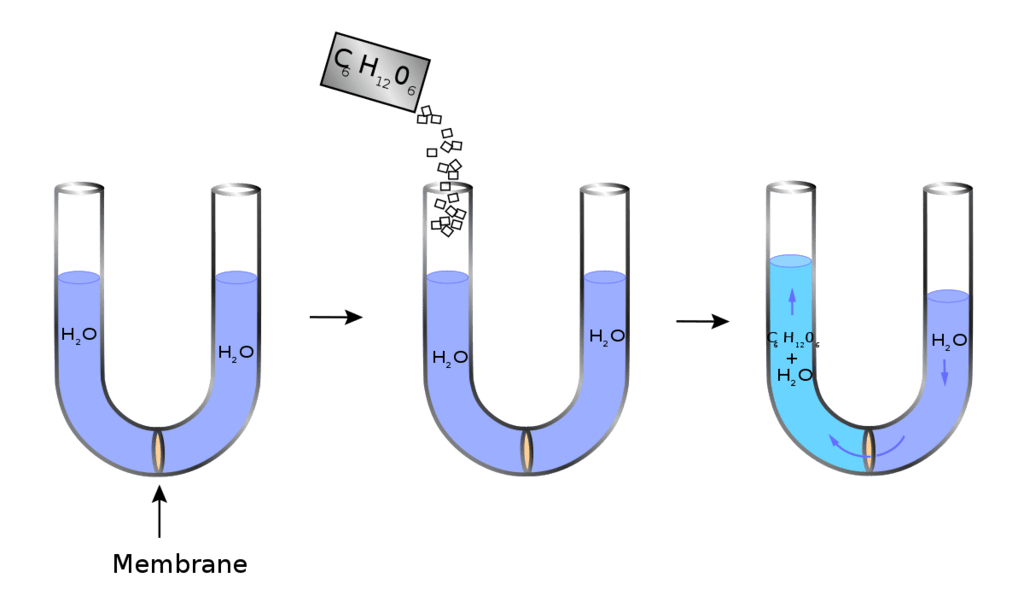The osmotic pressure p of a solution is related to the molar concentration of solute M as: π = MRT,

Where R is the gas constant and T is the absolute temperature.
Van’t Hoff equation for a dilute solution is similar to the ideal gas equation,
πV= nRT
Where π = osmotic pressure
R = gas constant
Some typical examples of osmosis and osmotic pressure are:
(i) The climbing of water up a tall tree from the soil.
(ii) Bursting of red-blood cells when placed in water.
(ii) Swelling of dry raisins when placed in water.

TYPES OF OSMOSIS

1. Exosmosis: This is the outward flow of water from a cell containing an aqueous solution through a semipermeable membrane, e.g. grape in NaCl solution.
2. Endosmosis: This is the inward flow of water into a cell containing an aqueous solution through a semipermeable membrane, e.g. grape in water.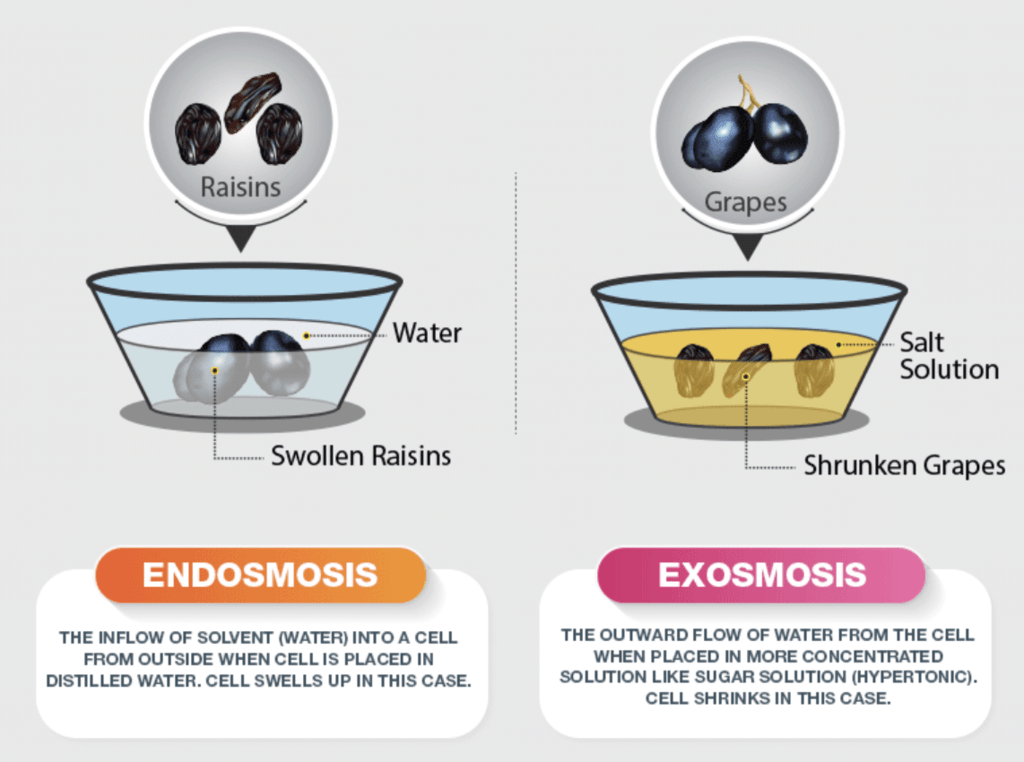Difference between Endosmosis and Exosmosis

Key concept: The semipermeable membranes allow a selective passage to only the solvent molecules. Cellophane, parchment paper and animal protein membranes are typical semipermeable membranes.

• The most frequently used semipermeable membrane in the laboratory is that of copper ferrocyanide, Cu2[Fe(CN)6] because it is very strong and can withstand very high pressure.
• Remember that the semipermeable membrane of Cu2[Fe(CN)6] does not work in non-aqueous solution because it gets dissolved in nonaqueous solvents.
• Another synthetic semipermeable membrane is that of calcium phosphate.
• Natural semipermeable membranes are cell walls, pig’s bladder, the skin surrounding white of an egg, the membrane surrounding RBCs etc.

The hydrostatic pressure set up as a result of osmosis is a measure of the osmotic pressure of the solution.
For instance, if the solution of density d rises to height h, then osmotic pressure is expressed as π = h × d × g, where g is the acceleration due to gravity.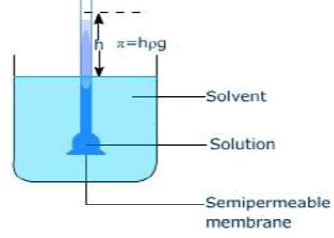TONICITY

The ability of an extracellular solution to make water move into or out of a cell by osmosis is known as its tonicity.

Three terms—hypertonic, hypotonic, and isotonic—are used to describe whether a solution will cause water to move into or out of a cell:

Isotonic Solution

A pair of solutions having the same osmotic pressure are known as isotonic solutions.

• If two such solutions are separated by a semipermeable membrane, there will be a transference of solvent from one solution to the other.
• Isotonic solutions have the same concentration.
• 0.85% NaCl solutions are found to be isotonic with blood, while 0.9%  NaCl solution isotonic with human RBCs.
For isotonic solution, π1 = π2
Or C= C⇒ n1/V1 = n2/V2
or W1/m1V1 = W2/m2V2

Hypertonic Solution

If a cell is placed in a hypertonic solution, there will be a net flow of water out of the cell, and the cell will lose volume. A solution will be hypertonic to a cell if its solute concentration is higher than that inside the cell, and the solutes cannot cross the membrane.

When placed in hypertonic solutions, the fluid from the plant cells comes out and thus the cells contract in size (plasmolysis). When excess fertilizers (like urea) are applied, plasmolysis takes place and plants dry up (wilt).

Hypotonic Solution

If a cell is placed in a hypotonic solution, there will be a net flow of water into the cell, and the cell will gain volume. If the solute concentration outside the cell is lower than inside the cell, and the solutes cannot cross the membrane, then that solution is hypotonic to the cell. When placed in water or hypotonic solutions, cell swell and burst (haemolysis).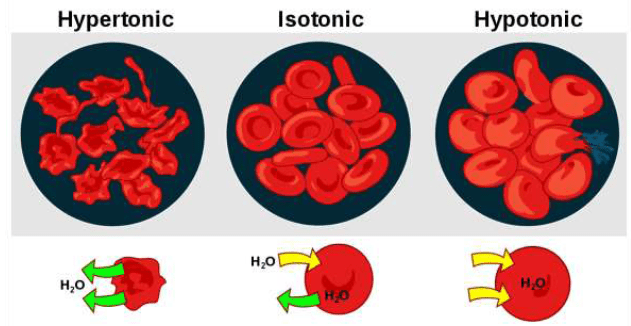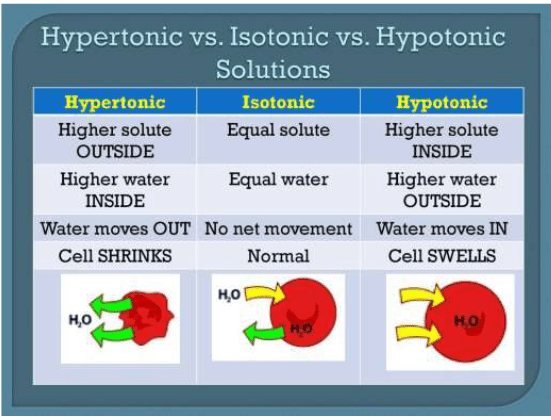COMPARISON BETWEEN DIFFUSION AND OSMOSIS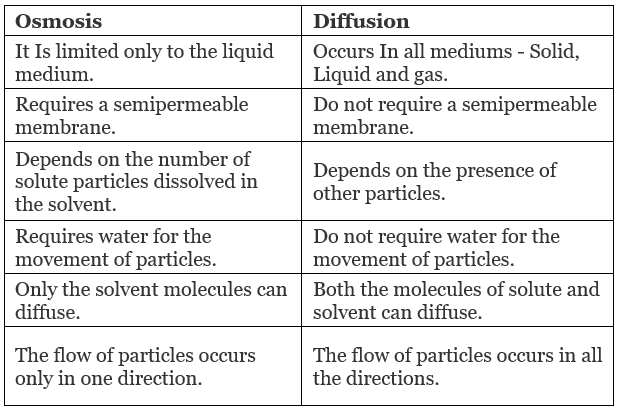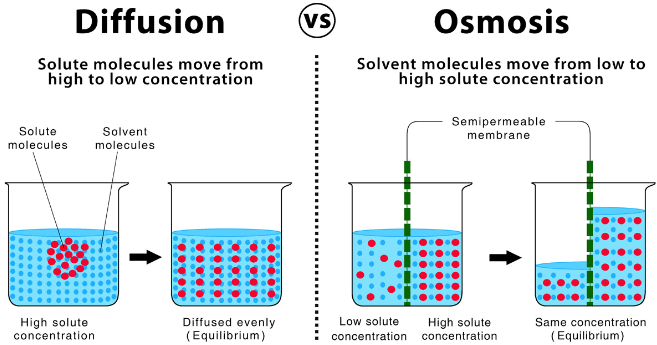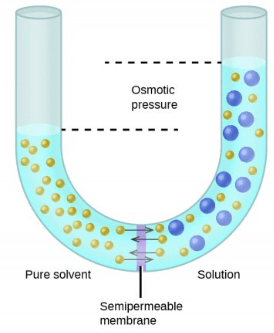Difference between Osmotic Pressure and Vapour Pressure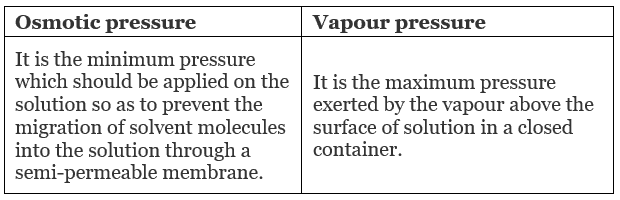Example 1. Calculate the osmotic pressure and vapour pressure of 0.6% aqueous solution of a non-volatile, non-electrolyte solute, urea (NH2CONH2) at 25˚C. The vapour pressure of pure water at 25˚C is 24 mm Hg. Take densities to be 1 g mL-1 and assume ideal behaviour of the solution. Gas constant, R = 0.082 L atm. mol-1 K-1.
Key concept: Please refer to difference between osmotic pressure and vapour pressure while solving this illustration.
Solution.
Concentration of urea solution = 0.6%
This means, solution contains 0.6 g of urea per 100 g, and hence 100 mL of the solution,
So,
Mass of urea per litre of solution
= 0.6/100 × 100 = 6 g
Molar mass of urea, M = 60 g/mol
Concentration of urea in solution
= 6/60 mol/L = 0.1 mol/L

Example 2. At 25˚C, the osmotic pressure of human blood due to the pressure of various solutes in the blood is 7.65 atm. Assuming that molarity and molality are almost same, calculate the freezing point of blood. Kf = 1.86 K kg/mol.
Solution.
According to the Van't Hoff equation,
p = CRT
c = 7.65/(0.082)(298) = 0.313 mol/L
Since molarity and molality are same,
ΔTf = Kfm
ΔTf = 1.86 × 0.313 = 0.582
Freezing point of blood is - 0.582˚C.

Example 3. At 27°C, 36 g of glucose per litre has an osmotic pressure of 4.92 atm. If the osmotic pressure of solution is 1.5 atm at the same temperature, what should be its concentration?
Solution.
Given that,
π= 4.92 atm, π2 = 1.5 atm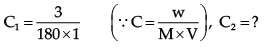π1V1 = n1RT1
π2V2 = n2RT2
At same temperature,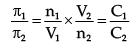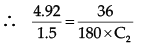∴ C2 = 0.061 mol/litre

Example 4. At 25°C, a solution containing 0.2 g of polyisobutylene in 100 mL of benzene developed a rise of 2.5 mm at osmotic equilibrium. Calculate the molecular weight of polyisobutylene if the density of the solution is 0.88 g/mL.
Solution.
Height developed = 2.4mm
Osmotic pressure = h.d.g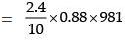207.187 dyne cm−2
Now πV = nRT
207.187 × 100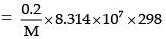(R in erg; V in mL, using CGS system)
M = 2.39

Try yourself!

Try yourself:A pair of solution bears the same osmotic pressure. What is this pair of solutions called?

Try yourself:A cell with lots of salt inside it is placed in a vessel containing just water. Which process takes place?

Try yourself:What is a necessary condition for osmosis to take place?

Try yourself:Which is the most appropriate method for determining the molar masses of biomolecules?

What is Reverse Osmosis (RO)?

RO is a process in which a large pressure is applied to the solution side so as to overcome the osmotic pressure.

This pushes the pure solvent under pressure, out of the solution through the semi-permeable membrane. This process finds a number of practical applications. Some of them are the purification of drinking water, removal of salt from water molecules, removal of effluents from water, etc.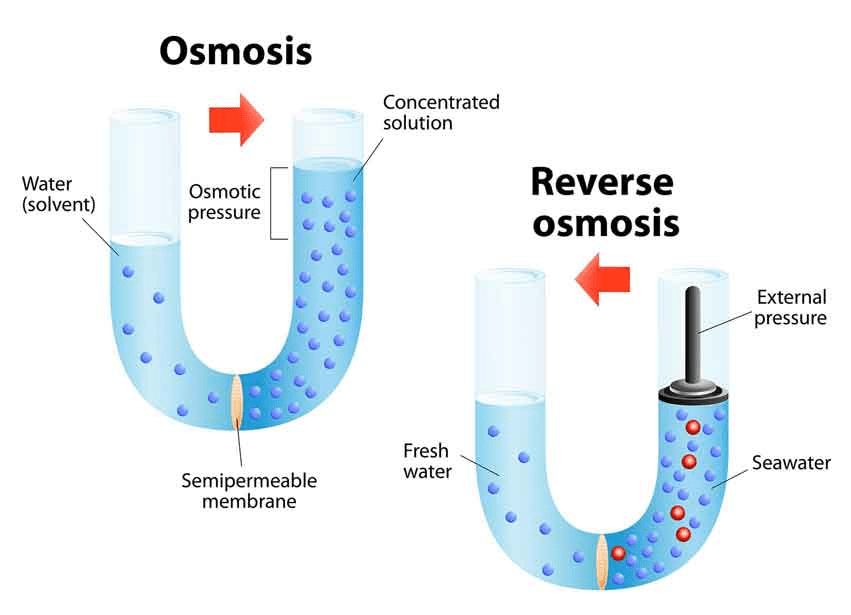Water Purification

RO is considered one of the most important water purification technologies. The contaminants present in water are removed by pushing water under pressure through a semi-permeable membrane. Pure water is thus squeezed out which is fit for drinking. RO is also used in the desalinization of seawater. RO is a reliable source for obtaining potable water.

• The process eliminates dissolved and suspended impurities, even bacteria.
• The membrane is small enough to allow the solvent molecules to pass but does not allow large ions or molecules to pass through its pores.
• Polymer membranes are used for the desalinization of seawater.
• Cellulose acetate is often used as a semi-permeable membrane. It allows water molecules to pass but is impermeable to impurities.
• It finds application in military, wastewater purification, food industry, landfill leachate purification, etc.
Offer running on EduRev: Apply code STAYHOME200 to get INR 200 off on our premium plan EduRev Infinity!

## Chemistry for JEE

196 videos|450 docs|369 tests

,

,

,

,

,

,

,

,

,

,

,

,

,

,

,

,

,

,

,

,

,

;